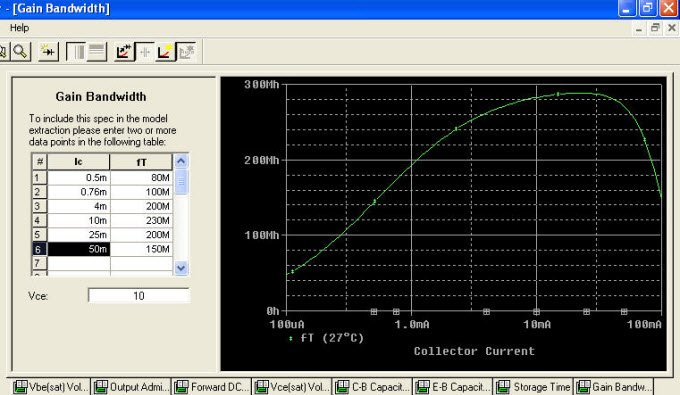# SPICE modeling of a BJT from Datasheet

Let’s check on Fixed to set the data.We have already estimated TF = 530ps. Then we can enter this value and fix it. Remain to be determined XTF, VTF, ITF.

If we input data couples of values from the graphic:We can see that the extraction of parameters leads to unreliable values because the graphic obtained is very far from the datasheet one:Set XTF, VTF, ITF to their default values (10, 10, 1), we’ll see that the curve starts to match datasheet curve:With a few attempts, we can have a good correspondence with the curve of the gain with the following values:TF=670ps, XTF= 150, VTF = 10, ITF1 = 1:finally, the model BC548A has the following expression:

.model BC548A NPN( Is=8.172f Xti=3 Eg=1.11 Vaf=139.172 Bf=212.95 +Ise=62.50f

+ Ne=1.37 Ikf=208.44m Nk=.839 Xtb=0 Br=0.608 Isc=13.68f Nc=1.80

+ Ikr=2.152 Rc=.86 Cjc=3.968p Mjc=.316 Vjc=.833 Fc=.5

+ Cje=6.808p Mje=.477 Vje=1.319 Tr=10n Tf=670p Itf=1 Xtf=150

+ Vtf=10)

Posted in PSpice Modeling from Datasheet and tagged , , , .